# 🌊🌈关于内容：

## 7.3.2_input 元素的其他属性

### (1) autofocus 属性

<!doctype html>
<html>
<meta charset="utf-8">
<title>autofocus属性的使用</title>
<body>

<form action="#" method="get">

<input type="text" name="user_name" autocomplete="off" autofocus="autofocus"/>
<br/>
<input type="submit" value="提交" />
</form>

</body>
</html>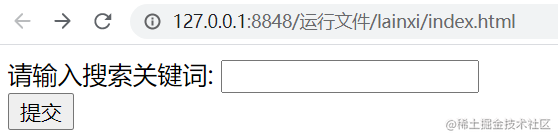### (2) form 属性

HTML5 中的 form 属性，可以把表单内的子元素写在页面中的任一位置， 只需为这个元素指定 form 属性并设置属性值为该表的 id 即可。此外，form 属性还允许规定一个表单控件属于多个表单。

<!doctype html>
<html>
<meta charset="utf-8">
<title>autofocus 属性的使用</title>
<body>

<form action="#" method="get" id="user_form">

<input type="text" name="first_name"/>
<input type="submit" value="提交" />
</form>

<p>下面的输入框在form元素外，但因为指定了form属性为表单的id,所以该输入框仍然属于表单的一部分。</p>

<input type="text" name="last_name" form="user_form"/><br/>

</body>
</html>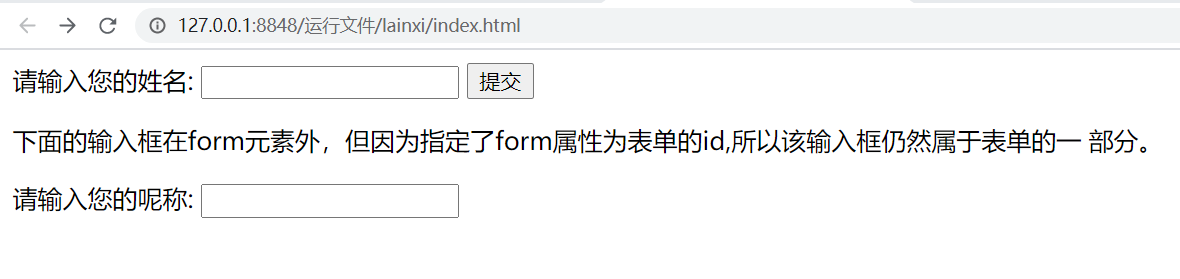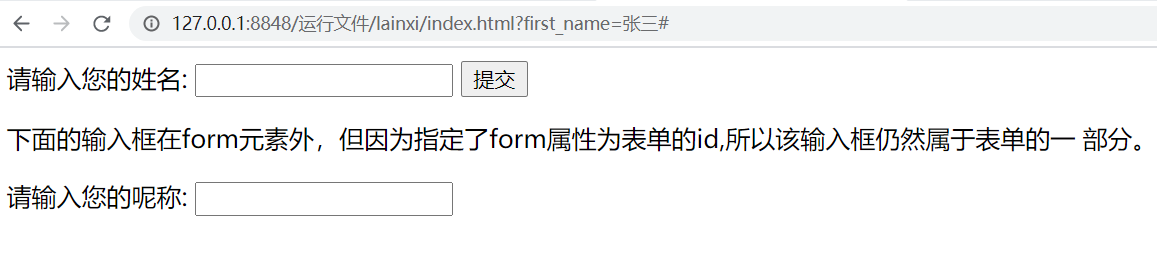• 注意:
_
form 属性适用于所有的 input 输入类型。在使用时，只需引用所属表单的 id 即可。

### (3) list 属性

<!doctype html>
<html>
<meta charset="utf-8">
<title>list 属性的使用</title>
<body>

<form action="#" method="get">

<input type="url" list="url_list" name="weburl"/>
<datalist id="url_list">
<option label="新浪" value="http://www.sina.com.cn"></option>
<option label="搜狐" value="http://www.sohu.com"></option>
<option label="阿ken的博客" value="https://blog.csdn.net/kenken_"></option>
</datalist>
<input type="submit" value="提交"/>
</form>

</body>
</html>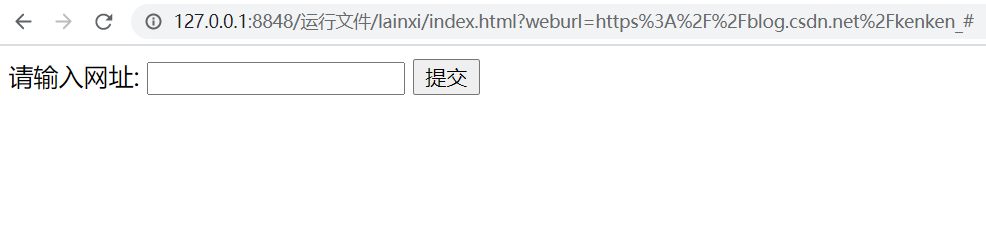上述案例中，分别向表单中添加 input 和 datalist 元素，并且将 < input /> 元素的 list 属性指定为 datalist 元素的 id 值。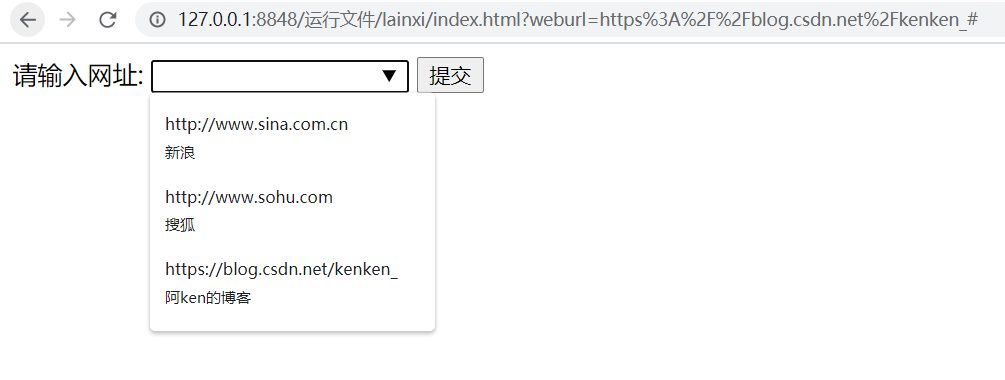### (4) multiple 属性

multiple 属性指定输入框可以选择多个值，该属性适用于 email 和 file 类型的 input 元素。muliple 属性用于 email 类型的 input 元素时，表示可以向文本框中输入多个 E-mail 地址，多个地址之间通过逗号隔开; multiple 属性用于 file 类型的 input 元素时，表示可以选择多个文件。

<!doctype html>
<html>
<meta charset="utf-8">
<title>multiple 属性的使用</title>
<body>

<form action="#" method="get">

<input type="email" name="myemal1" multiple="multiple"/>&nbsp;&nbsp; (如果电子邮箱有多个，请使用逗号分隔)
<br/><br/>

<input type="file" name="selfile" multiple="multiple"/>
<br/><br/>
<input type="submit" value="提交"/>
</form>

</body>
</html>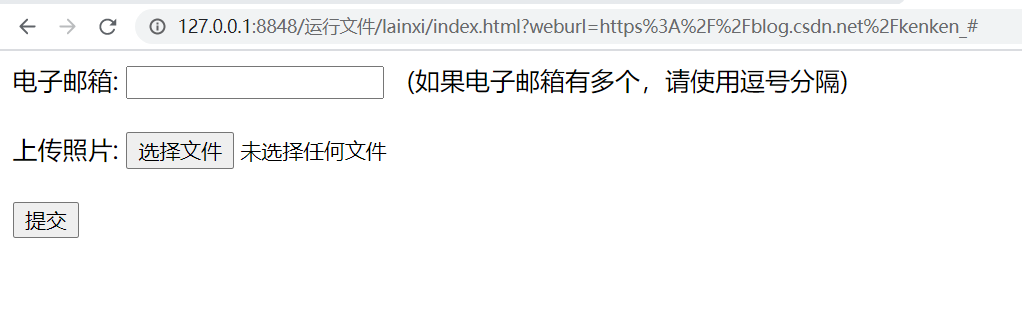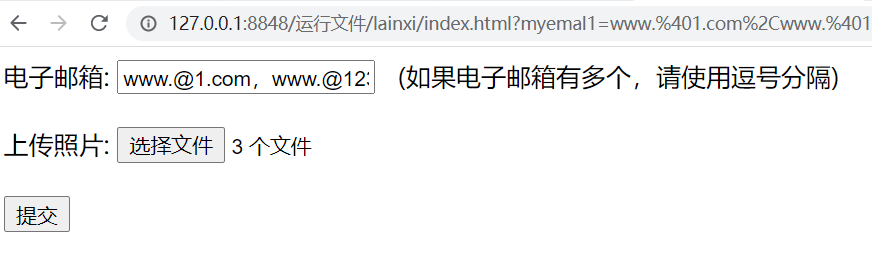### (5) min、 max和step属性

HTML5 中的 min、max 和 step 属性用于为包含数字或日期的 input 输入类型规定限值，也就是给这些类型的输入框加一个数值的约束， 适用于date、pickers、 number 和 range 标签。具体属性说明如下：

• max: 规定输入框所允许的最大输入值。
• min: 规定输入框所允许的最小输入值。
• step: 为输入框规定合法的数字间隔，如果不设置，默认值是1。

### (6) pattern 属性

pattern 属性用于验证 input 类型输入框中，用户输入的内容是否与所定义的正则表达式相匹配。pattern 属性适用于的类型是: text、search、url、tel、email 和 password 的 < input/> 标记。

^ [0-9]*$数字 ^\d{ n }$n 位的数字
^\d{ n, }$至少 n 位的数字 ^\d( m,n )$m - n 位的数字
^(0竖线[1-9] [0-9] *)$零和非零开头的数字 ^([1-9][0-9]*)+(.[0-9] { 1,2 } ?$非零开头的最多带两位小数的数字
^(-竖线+)?\d+(.\d+)?$正数、负数和小数 ^\d+$或 ^ [1-9]\d*竖线0$非负整数 ^-[1-9]\d*竖线0$或 ^((-\d+)竖线(0+))$非正整数 ^ [\u4e00-\u9fa5] { 0, }$汉字
^ [A-Za-z0-9]+$或^ [A-Za-z0-9]{ 4,40 }$英文和数字
^ [A-Za-z]+$由26个英文字母组成的字符串 ^ [A-Za-z0-9]+$由数字和26个英文字母组成的字符串
^ \w+$或^\w{ 3,20 }$由数字、26个英文字母或者下划线组成的字符串
^\u4E00-\u9FA5A-Za-z0-9_ ]+$中文、英文、数字包括下划线 ^\w+([-+.]\w+)* @\w+([-.]\w+)* \ .\w+([-.])\w+)*$E-mail 地址
(a-zA-z]+://[ ^\s ]* 或^http://([\w-]+.)+[\w-]+(/[\w-./?%&=]* )?$URL地址 ^\d{ 15 }竖线\d{ 18 }$身份证号(15位、18位数字)
^([0-9]){ 7,18 }(x竖线X)?$或 ^ \d{ 8,18 }竖线[0-9x]{ 8,18 }竖线[0-9X]{ 8,18 }?$以数字、字母x结尾的短身份证号码
^ [a-zA-Z] [ a-zA-Z0-9_]{ 4,15 }$账号是否合法(字母开头，允许5~16字节，允许字母数字下划线) ^ [a-zA-Z]\w{ 5,17 }$密码(以字母开头，长度为6~18.只能包含字母、数字和下划线)年

<!doctype html>
<html>
<meta charset="utf-8">
<title>pattern 属性</title>
<body>

<form action="#" method="get">

<input type="text" name="username" pattern="^ [a-zA-z] [a-zA-Z0-9_] { 4,15 }$" />(以字母开头，允许 5 ~ 16 字节，允许字母数字下划线) <br/> 密&nbsp;&nbsp;&nbsp;&nbsp;码: <input type="paasword" name="pwd" pattern=" ^[a-zA- Z]\w{ 5,17 }$" /> (以字母开头，长度在6~18，只能包含字母、数字和下划线) <br/>

<input type="text" name="mycard" pattern="^\d{ 15 }|\d{ 18 }$" /> (15位、18位数字) <br/> E-mail 地址: <input type="email" name="myemail" pattern="^\w+([-+.J]\w+)*@\w+([-.]\ W+)*\.\w+([-.]\w+)*$"/>
<input type="submit" value="提交"/>
</form>
</body>
</html>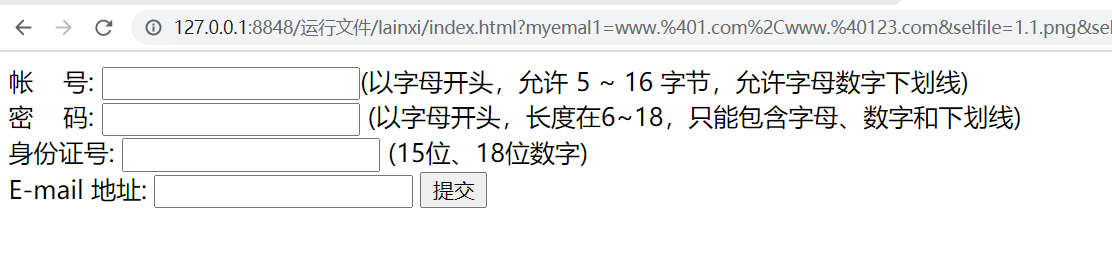### (7) placeholder 属性

placeholder 属性用于为 input 类型的输入柜提供相关提示信息，以描述输入框期待用户输入何种内容。在输入框为空时显式出现，而当输入框获得焦点时则会消失。

<!doctype html>
<html>
<meta charset="utf-8">
<title>placeholder 属性</title>
<body>

<form action="#" method="get">

<input type="text" name="code" pattern="[0-9]{ 6 }" placeholder="请输入6位数的邮政编码"/>
<input type="submit" value="提交"/>
</form>

</body>
</html>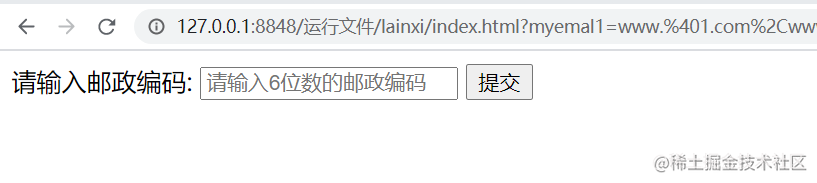• 注意:
_
placeholder 属性适用于 type 属性值为 text、search、url、tel、email 及 password 的< input/>标记。

### (8) required 属性

HTML5 中的输入类型，不会自动判断用户是否在输入框中输入了内容，如果开发者要求输入框中的内容是必须填写的，那么需要为 input 元素指定 required 属性。required 属性用于规定输入框填写的内容不能为空，否则不允许用户提交表单。

<!doctype html>
<html>
<meta charset="utf-8">
<title>required 属性</title>
<body>

<form action="#" method="get">

<input type="text" name="user_name" required=" required"/>
<input type="submit" value="提交"/>
</form>

</body>
</html>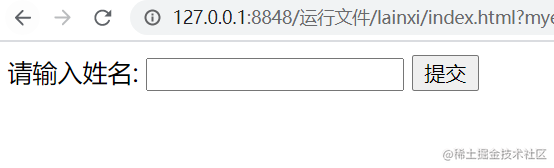Peace